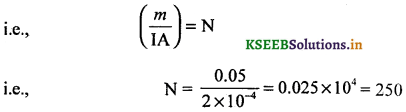# 2nd PUC Physics Question Bank Chapter 5 Magnetism and Matter

Students can Download 2nd PUC Physics Chapter 5 Magnetism and Matter Questions and Answers, Notes Pdf, 2nd PUC Physics Question Bank with Answers helps you to revise the complete Karnataka State Board Syllabus and to clear all their doubts, score well in final exams.

## Karnataka 2nd PUC Physics Question Bank Chapter 5 Magnetism and Matter

### 2nd PUC Physics Magnetism and Matter One Marks Questions and Answers

Question 1.
What is meant by Iodestone or loadstone?
Loadstone is the name given to the naturally occurring ore of iron -magnetite.

Question 2.
Mention any one country to have used this magnet for technology long ago.
China. Chinese texts dating to 400 BC have recorded the usage of magnets as magnetic needles for navigation on ships.

Question 3.
Is it possible to make magnets out of iron and its alloys?
Yes. e.g.: Alnico an alloy of aluminum, nickel and cobalt is a ferromagnetic substance.

Question 4.
Can the north or the south pole of a magnet be isolated?
No. magnetic monopoles do not exist.

Question 5.
Give any one cause for terrestrial magnetism.
The dynamic condition of iron and nickel at the core of the earth is responsible for terrestrial magnetism.Question 6.
What does magnetic flux refer to?
Magnetic flux refers to the total number of magnetic lines of force around a current carrying conductor.

Question 7.
Write the expression for magnetic dipole moment, due to a current loop.
Magnetic moment $$\vec{m}$$ = NI$$\vec{A}$$, where, $$\vec{A}$$ represents the area vector.

Question 8.
Define the term magnetic moment with respect to a magnet.
Magnetic moment is the product of pole strength of a bar magnet and the distance between the poles of the magnet.

Question 9.
Write the SI unit of magnetic dipole moment.
The SI unit of magnetic dipole moment is Am2.

Question 10.
Give the SI unit of pole strength.
The SI unit of magnetic pole strength is Am.

Question 11.
Write the dimensional formula for $$\frac{I}{m B}$$ where symbols have their usual meaning.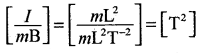Question 12.
Does a bar magnet exert a torque on itself due to its own field?
No.Question 13.
What is the pole named near the geographic north pole of the earth and near the geographic south pole of the earth?
North magnetic pole and south magnetic pole respectively.

Question 14.
What is a geographic meridian?
A vertical plane containing the longitude circle and the axis of rotation of the earth is called geographic meridian.

Question 15.
What is meant by a magnetic meridian?
A vertical plane containing the axis of a freely suspended magnet at a given place is called magnetic meridian (earth’s horizontal component of the field).

Question 16.
What is meant by magnetic declination? (March 2014)
The angle between the magnetic meridian and the geographic meridian is known as the magnetic declination.

Question 17.
What is meant by magnetic inclination (or angle of dip)? (March 2014)
The angle between the earth’s magnetic meridian (horizontal component of earth’s field) and the earth’s total magnetic field is known as the angle of dip.

Question 18.
Define intensity of magnetization.
The net magnetic moment acquired per unit volume of a specimen is known as the intensity of magnetization.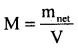Question 19.
Write the unit of intensity of magnetisation.
The SI unit of intensity of magnetization is Am-1

Question 20.
Define magnetic permeability of a substance.
Magnetic permeability of a substance may be defined as the ratio of magnetic flux density (induction) to the magnetising field.
µ = $$\frac{B}{H}$$Question 21.
Mention the SI unit of magnetising field.
The SI unit of magnetising field is Am-1.

Question 22.
Give the expression for the magnetic filed contributed by the material core.
Bm = µ0M. Where, M – intensity of magnetisation.

Question 23.
What are diamagnetic substances?
Diamagnetic substances are those substances which show the tendency of moving away from the stronger magnetic field towards the weaker magnetic field.

Question 24.
Give an example for a diamagnetic substance.
Bismuth (other examples are copper, water, nitrogen, diamond, gold, lead, mercury, silver, silicon).

Question 25.
What are paramagnetic substances?
Paramagnetic substances are those substances which show the tendency of moving away from a weaker field towards the stronger magnetic field.

Question 26.
Give an example for a paramagnetic substance. (March 2014)
Aluminum. (Copper chloride, Calcium, Chromium, Bromium, Liquid Oxygen).

Question 27.
What are ferromagnetic substances?
Ferromagnetic substances are those substances which show a stronger tendency to move from a weaker magnetic region toward a stronger magnetic field region than paramagnetic substances.

Question 28.
Give an example for a ferromagnetic substance.
Iron.
(other examples are alloys of iron, alloy of alnico, salts of iron).

Question 29.
How does magnetic susceptibility of a diamagnetic substance depend on the absolute temperature?
Magnetic susceptibility of a diamagnetic substance is independent of absolute temperature.

Question 30.
Mention the range of relative magnetic permeability of a diamagnetic substance.
0 ≤ µr< 1.Question 31.
State Curie’s law for a paramagnetic substance.
Magnetic susceptibility of a paramagnetic substance is inversely proportional to the absolute temperature.

Question 32.
Give the range of magnetic permeability for a paramagnetic substance.
‘µr‘ is slightly more than one. Mathematically for a small positive number ‘ε’, 1 < µr< 1 + ε.

Question 33.
Give the range of magnetic permeability for a ferromagnetic substance.
Magnetic permeability µr >> 1.

Question 34.
Define curie temperature.
The temperature of transition from ferromagnetic to paramagnetism is called the curie temperature.

Question 35.
State Curie-Weiss law for a ferromagnetic substance.
Magnetic susceptibility of a ferromagnetic substance is inversely proportional to the absolute temperature above the critical temperature, expressed in absolute scale of temperature.
Note : TC(K) for cobalt, iron, nickel and gadolinium are 1394 K, 1043K, 63IK and 317K respectively.

Question 36.
Define the term residual magnetism.
The quantity of magnetism present in a specimen after the removal of the magnetizing field is known as the residual magnetism.

Question 37.
Define the term retentivity or remanence.
The property of a material to retain the magnetism even after the removal of the magnetising field is known as retentivity.

Question 38.
What is meant by a coercive field?
The negative or reverse magnetising field required to remove the residual magnetism completely from a specimen, is known as a coercive field.

Question 39.
What is meant by coercivity?
Coercivity refers to the property of a material which shows the tendency of opposition to the removal of residual magnetism in the material.Question 40.
What is hysteresis?
The phenomenon of lagging of magnetic flux density or induction behind the magnetising field is known as hysteresis.

Question 41.
Suggest a material that Can be used to make a permanent magnet.
Steel, alnico, cobalt steel, and ticonal.

Question 42.
Name the material that can be used to make electromagnets.
Soft iron (which has a high permeability and low retentivity).

Question 43.
Why do diamagnetic substances repel away from a strong magnetic field.
A diamagnetic substance develops a net magnetic moment in a direction opposite to that of the applied field and hence repels away from a strong magnetic field.

Question 44.
If a bar magnet is placed in a uniform magnetic field then what will be the net force experienced by it?
Zero.

Question 45.
What is the value of P.E of a magnetic dipole whose orientation is 90° with respect to the magnetic flux density?
Zero.

Question 46.
Mention the value of $$\oint \overrightarrow{\mathbf{B}} \cdot \mathrm{d} \overrightarrow{\mathbf{S}}$$ through any closed surface.
Zero.

Question 47.
How would you relate p and pr? (where p is magnetic permeability and p relative magnetic permeability)
µ = µoµr where µo = absolute magnetic permeability for free space (4π × 10-7 Hm1)

Question 48.
How are magnetic susceptibility and relative magnetic permeability (p ) related?
µr = 1 + χ.Question 49.
Why do we say that diamagnetism is universal.
Diamagnetism is present in all the materials but is weak and hard to detect. Hence diamagnetism is universal.

Question 50.
What are ceramics?
Ceramics are specially treated barium iron oxides. These are also referred to as ferrites.

Question 51.
Would the maximum possible magnetisation of a paramagnetic substance be of the same order of magnitude as the magnetisation of a ferromagnet?
yes. But saturation level requires impractically high magnetising fields.

Question 52.
What is meant by magnetic equator?
Magnetic equator or aclinal line is a circular plane at a point on which the angle of dip is zero.

Question 53.
Give the expression for magnetic field at a point on the axis of a short magnetic dipole.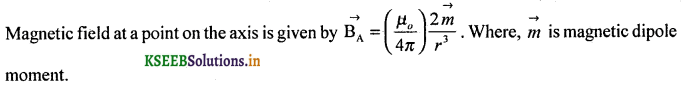Question 54.
Give the expression for magnetic field at a point on the equatorial line of a short magnetic dipole.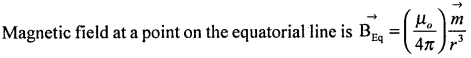Question 55.
Give a relation between magnetic field as a point on the axis and equatorial point of short magnetic dipole.Question 56.
What is meant by solar wind?
Solar wind is a stream of charged particles emitted by the sun, some of which flow towards Earth.
Note: The charged particles emitted by the sun and those moving towards the Earth are trapped in the ionosphere and show helical motion before advancing towards the south pole and the north pole. These charged particles interact with N2 and 02 molecules and cause a spectacular display of colours in the sky at a height from 500 km to 650 km above the surface of the earth. These are called Auroras. (The one which is observed in Australia is called Aurora Australis and the one which is observed near Canada is called Aurora Borealis).

Question 57.
Name a device that shows the direction of horizontal component of a magnetic field.
Compass box (a device in which the compass needle is pivoted to rotate in the horizontal plane).

Question 58.
Draw magnetic field lines around, a bar magnet. (March 2014)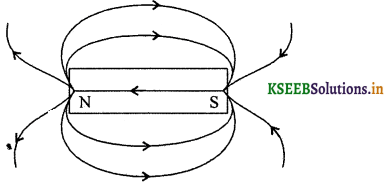### 2nd PUC Physics Magnetism and Matter Two Marks Questions and Answers

Question 1.
What are electromagnets?
When ferromagnetic substances are subjected to a varying electric field, they acquire magnetism. In the absence of the electric field, the FMS gets demagnetised. These are called electromagnets.

Question 2.
Write the relation between BR, BH and Bv along with an appropriate diagram.B2R = B2H + B2v
So that BR = $$\sqrt{B_{H}^{2}+B_{V}^{2}}$$

Question 3.
What do magnetic lines of force represent?
These are imaginary lines drawn along the path of deflection of a magnetic needle in a given magnetic field, representing magnetic lines of force which help in determining the magnetic flux and hence the magnetic flux density.

Question 4.
Why is that any two magnetic lines of force do not intersect with each other?
At the point of intersection of two lines of force, one should expect to draw two tangents and two directions of the magnetic field which is against the law of nature.Question 5.
How are the magnetic lines of force aligned in an uniform magnetic field?
The magnetic lines of force will be parallel to each other so that throughout the domain, the magnitude of the magnetic flux density and direction remain the same.

Question 6.
Say whether the magnetic dipole moment is a scalar or a vector quantity and give its, direction.
The magnetic dipole moment is a vector physical quantity. The direction of magnetic dipole moment points from magnetic south pole to north pole.

Question 7.
Give the expression for the magnitude of magnetic flux density along the axis of a solenoid in terms of magnetic moment.
Give the meaning of the symbols used.
The magnitude of magnetic flux density at a point along the axis of a solenoid is given by,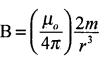where, m = magnetic moment of the solenoid.
= N(2l) I (πa2),
= 2πNIla2
where ‘a’ is radius of the solenoid, N-number of turns, I – current and 2l – length of the solenoid.

Question 8.
Give the expression for torque acting on a magnetic dipole placed in an uniform magnetic field and give the meaning of the symbols.
The torque acting on a magnetic dipole (magnetic needle) is given by $$\vec{τ}$$ = $$\vec{m}$$ x $$\vec{B}$$.
Note : |$$\vec{τ}$$| = mB sin θ where ‘θ’ is the angle between $$\vec{m}$$ and $$\vec{B}$$ and m = magnetic moment.

Question 9.
Give the expression for magnetic potential energy and the meaning of the symbols.
Magnetic P.E., Um = ∫τ(θ)dθ.
= -mB cos θ
= –$$\vec{m}$$.$$\vec{B}$$.
Where τ = mB sin θ. τ = torque
m = magnetic moment
θ = angle between the axis of the needle and magnetic field lines.Question 10.
Give the expression for period of oscillation of a magnetic dipole (magnetic needle) in an uniform magnetic field and the meaning of the symbols.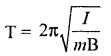where I represents the moment of inertia of a magnet, m the magnetic dipole moment, B the magnetic flux density.

Question 11.
State Gauss’s law in magnetism and write the same in the mathematical form.
The net magnetic flux through any closed surface is zero.Question 12.
Magnetic field arises due to charges in motion. Can a system have magnetic moments even though its net charge is zero? Explain.
Yes. The average of the magnetic moments due to various current loops may not be zero.

Question 13.
Give the location of earth’s magnetic dipole.
The location of the north magnetic pole is at a latitude of 79.74°N and a longitude of 71.8°W (North Canada) and the magnetic south pole is at 79.74°S, 108.22° E in the Antarctica.

Question 14.
Define magnetic susceptibility of a substance. Mention the SI unit of susceptibility. (March 2014)
Magnetic susceptibility of a substance may be defined as the ratio of intensity of magnetisation (M) to the magnetising field (H).
χ = $$\left(\frac{M}{H}\right)$$
Susceptibility has no unit.Question 15.
Give the expression for total magnetic flux associated with the magnetic material subjected to the magnetising Held.
B = µ0 (H + M).
B = µ0(H + χH).
i.e., B = µ0H (1 + χ) put µr = 1 + χ.
Hence, B = µ0µrH = µH, µoH = magnetic flux due to external field,
µχH- magnetic flux due to internal field.

Question 16.
What are permanent magnets? Give any one practical application of permanent magnets.
Substances, which at room temperature, retain their ferromagnetic property for a long period of time are called permanent magnets. Permanent magnets are used is moving coil galvanometers, dynamos and motors.

Question 17.
What are magnetic elements of the Earth?
Magnetic elements determine the characteristics of terrestrial magnetism. Earth’s total magnetic field, including its components, magnetic declination and magnetic inclination are magnetic elements of the Earth.

Question 18.
Why does a paramagnetic sample display greater magnetisation (for the same magnetising field) when cooled?
Magnetic dipoles will maintain their alignment at lower temperatures. However due to thermal motion, at higher temperature there is disruption of the alignment of dipoles.

Question 19.
What are horizontal and vertical components of earth’s total magnetic field? (March – 2014)
The axis of a freely suspended magnet in a horizontal plane is called magnetic meridian or horizontal components of earth’s field.
The vertical component of earth’s total magnetic field with respect to the axis of a freely suspended magnet is called vertical components of earth’s field.### 2nd PUC Physics Magnetism and Matter Three Marks Questions and Answers

Question 1.
Mention the range of magnetic susceptibility for
(a) Diamagnetic substances (DMS)
(b) Paramagnetic substances (PMS)
(c) Ferromagnetic substances (FMS).
(a) For DMS, x is very low and negative – 10-9 to -10-5.
e.g : For copper, χ is -9.8 × 10-6.

(b) For PMS, χ is very low but positive +10-6 to +10-4
e.g : For platinum, χ is +2.9 × 10-4.

(c) For FMS, χ >> 1, 101 to 10+6.
e.g : Supermalloy (Ni79%, Fe 16%, Mo5%) has χ = 106.

Question 2.
Explain the significance of narrow hysteresis loop with an example.
Magnetic (ferro) substances having narrow hysteresis loops lose less amount of heat per cycle. These substances are generally used as cores of chokes and transformers, e.g : Permalloy, an alloy of Fe and Ni shows a narrow hysteresis curve and hence used as the core material for chokes and transformers.

Question 3.
Give any three practical applications of electromagnets.
Electromagnets are used in electric bells, transducers like loud speakers and microphones telephone diaphragms and in certain types of motors and dynamos.
Giant electromagnets are used in cranes to lift machinery and bulk quantities of iron and steel.

Question 4.
If the horizontal component of Earth’s magnetic Held is 0.26G, then find the total magnetic field at which the dip angle is 60°.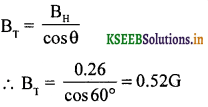Question 5.
If the total magnetic field at the place is 0.56 G, then calculate the vertical component of Earth’s magnetic field at which the dip angle is 30°.
BE = $$\frac{\mathrm{B}_{\mathrm{E}}}{\sin \theta}$$, BE – total Earth’s magnetic field
Bv = 0.56 × 1/2 = 0.28 G (1G = 10-4 T)Question 6.
Write any three known facts about magnetism.

• Like poles repel away from each other and unlike poles attract each other.
• North pole and South pole cannot be isolated.
• A freely suspended magnet aligns in the direction of north and south poles.

Question 7.
Write any three properties of magnetic field lines. (July 2014)
Magnetic lines of force act like a stretched elastic cord and this elastic property of lines of force explain why two magnetic like poles brought closer, should repel.

• Two magnetic lines of force do not intersect with each other.
• In the region of a strong magnetic field, magnetic flux will be very large.
• In the region of uniform magnetic field, magnetic lines of force are parallel to each other.

Question 8.
State and explain Gauss’s law in magnetism.The net magnetic flux passing normally outward through any closed Surface is zero.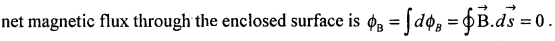This is because, the number of magnetic lines of force leaving the surface (+ΦB) will be equal to the number to magnetic lines of force entering the surface (- ΦB). ∴ Net Φ = +ΦB – ΦB = 0.

Question 9.
Draw a neat diagram to show the angle of dip and angle of declination.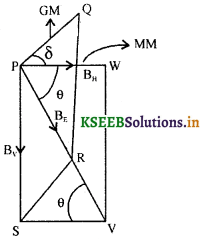GM – geographic meridian.
MM – magnetic meridian.
δ – angle of declination.
θ – angle of dip.
Along PW → BH – horizontal component field.
Along PS → Bv – vertical component of field.
BE → Earth’s total magnetic field.Question 10.
Calculate the value Of angle of dip corresponding to the place
(i) at which Bv = BE
(ii) at which BH = BE
(i) We know that Bv = BE sin θ
For Bv = BE, sin θ = 1, i.e., θ = 90° (atthe poles)

(ii) We know that BH = BE cos θ
For BH = BE, cos θ = 1, θ = 0° (at the magnetic equator)

Question 11.
Distinguish between diamagnetic and paramagnetic substances. (March 2015)Question 12.
Distinguish between paramagnetic and ferromagnetic substances. (July 2014)### 2nd PUC Physics Magnetism and Matter Five Marks Questions and Answers

Question 1.
Explain briefly how bar magnets act as equivalent solenoids.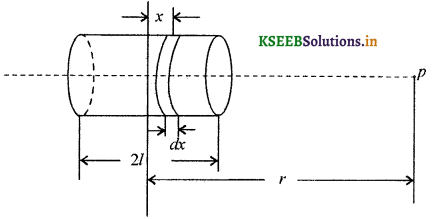The magnitude of magnetic field at a point ‘P’ due to a circular element of thickness ‘dx’ is given byThe magnitude of total magnetic field is given by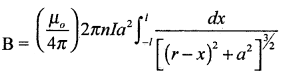This expression is similar to the magnetic field at a point on the axis of a short magnet. Therefore a bar magnet and a solenoid produce similar magnetic fields.

Question 2.
Obtain an expression for magnetic potential energy of a magnetic dipole placed in an uniform magnetic field.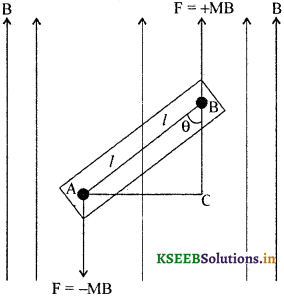Let M be the magnetic pole strength.
Let B be the uniform magnetic field.
Let NS represent magnetic dipole of length 2l.
Let θ be the angle between the magnetic axis and the magnetic field.
The magnetic force on the north pole is MB and that on the south pole is -MB.
The torque acting on the magnetic dipole = τ = MB × perpendicular distance between the two parallel forces.
From the figure sin θ = $$\frac{A C}{A B}$$. So that AC = AB sin θ – 2l sin θ.
Torque = τ = MB 2l sin θ. But magnetic dipole moment m = 2l M.
τ = mB sinθ. Work done by torque on the dipole = W = ∫τdθ
i. e., W = ∫mB sinθ dθ = -mB cosθ
This work done is stored in the form of potential energy. P.E. = -mB cosθ = $$\vec{m}$$.$$\vec{B}$$.Question 3.
What is meant by a hysteresis curve? Explain hysteresis curve. Give any one application of the study of hysteresis curve.
A curve obtained by plotting the magnetic induction along the ‘y’ axis and intensity of magnetisation along the x – axis is known as the hysteresis curve.

The area bounded inside the hysteresis curve determines the amount of work done by the process of magnetisation.

If the hysteresis loop is narrow then less heat will be dissipated by the specimen (Ferromagnetic substances). Substances having high retentivity, high permeability and large coercivity are used for manufacturing permanent magnets. Substances having narrow hysteresis loop are used as cores of transformers and chokes.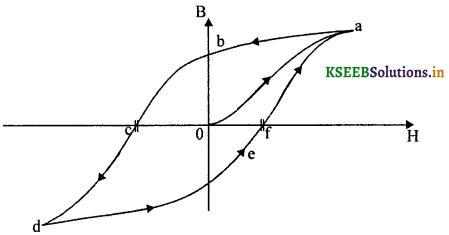### 2nd PUC Physics Magnetism and Matter List of Formulae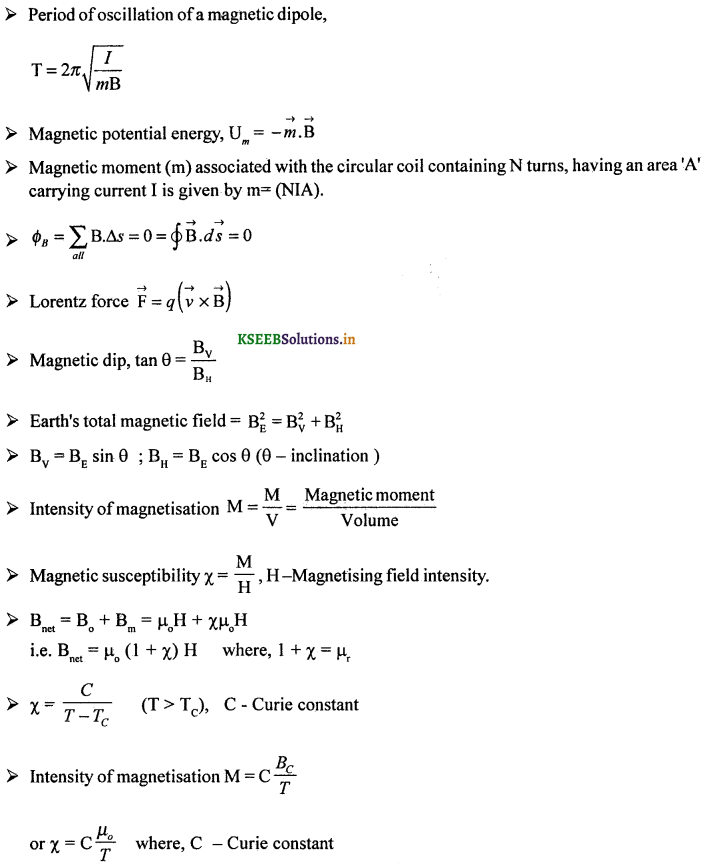### 2nd PUC Physics Magnetism and Matter Numericals and Solutions

Question 1.
For a short magnetic dipole of magnetic moment 0.5 Am2. Find the magnetic field at a point
(a) 1 m on the axis and from the centre of the magnet (dipole)
(b) 1 m on the equatorial line from the centre
(c) at an angle of 60° w.r. to the line joining the point and the centre of the dipole at distance 1 m.
We know that,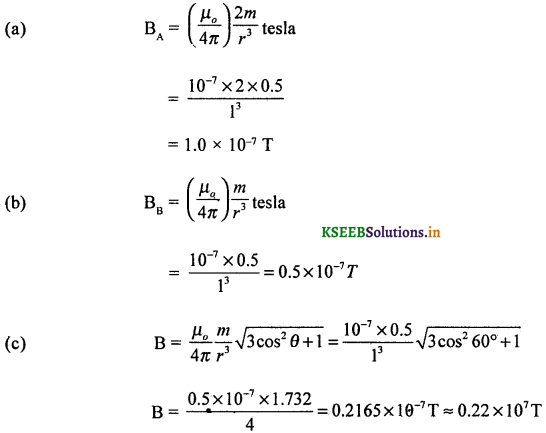Question 2.
A charge 2 µC is moving at an angle of 60° with respect to the magnetic field 0.2 T at a speed of 104 ms-1. If the electric field 103 Vm-1 is at right angles to the magnetic field, then find the net field.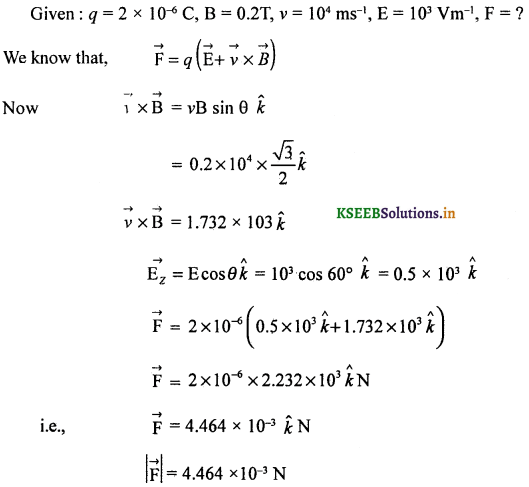Question 3.
A domain in a ferromagnetic substance is in the form of a cube of volume 10-18m3. Calculate the number of atoms present in the given volume and a hypothetical dipole moment and magnetisation of the domain (given density of the substance 7.9 × 103 kgm-3, molecular mass 55g / mole and dipole moment of each atom is 9.2 × 10-24 Am2).
Given volume of the cube = 10-18 m3
mass = (volume) (density)
= 10-18m3 × 7.9 × 103 kg m-3.
i.e., m = 7.9 × 10-15 kg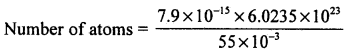= 0.865 × 1011
= 8.65 × 1010 atoms
Maximum dipole moment = (m)each N
= 9.27 × 10-24 × 8.65 × 1010
= 80.185 × 10-14 Am2
Intensity of magnetisation,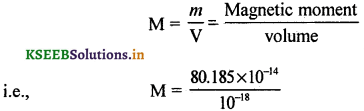= 80.185 × 104
= 8.018 × 105 Am2

Question 4.
A short bar magnet placed with its axis at 30° with uniform external magnetic field of 0.25 T, experiences a torque of magnitude equal to 4.5 × 10-2 Nm. What is the magnitude of the magnetic moment of the magnet?
θ = 30°, B = 0.25 T, τ = 4.5 × 10-2 Nm.
we know that, |$$\vec{τ}$$| = mB sin θ, sin 30° = 1/2i.e., m = 0.36 Am2 or J T-1
m = 0.36 Am2Question 5.
A short bar magnet of magnetic moment m = 0.32J T-1 is placed is a uniform magnetic field of 0.15T-1. If the bar magnetis free to rotate in the plane of the field, which orientation would correspond to its
(a) stable
(b) unstable equilibrium? What is the rotational energy of the magnet?
m = 0.32 J T-1, B = 0.15T
(a) $$\vec{m}$$ parallel to $$\vec{B}$$
U = -mB
= -0.32 × 0.15. J
= -0.048 J, for a stable equilibrium.

(b) $$\vec{m}$$ antiparallel to $$\vec{B}$$
W = + mB
i.e., W = 0.32 × 0.15 J = 0.048J, for an unstable equilibrium.
(c) Rotational energy of the magnet is zero.

Question 6.
A closely wound solenoid of 800 turns and of cross section 2.5 × 10-4 m2 carries a current of 3.0 A. Explain the sense in which the solenoid acts like a bar magnet. What is its associated magnetic moment?
Given : N = 800 turns, A = 2.5 × 10-4 m2, I = 3.0
Magnetic moment = NIA
= 800 × 2.5 × 10-4 × 3
= 60 × 10-2
= 0.60 Am-2 or JT-1 along the axis of the solenoid.Question 7.
A bar magnet of magnetic moment 1.5J T-1 lies aligned with the direction of a uniform magnetic field of 0.22 T.
(a) What is the amount of work required by an external torque to turn the magnet so as to align its magnetic moment
(i) Normal to the field direction
(ii) opposite to the field direction?
(b) What is the torque on the magnet is cases (i) and (ii)?
(a) Given: m = 1.5 J T-1, B = 0.22 T, θ = 0
Work done = -mB cosθ°
= -mB
= -1.5 × 0.22
= -0.33 J

(i) θ = 90°, cos 90° = 0
∴ W = Δ PE
= 0 + 0.33
= + 0.33 J

(ii) θ = 180°, cos 180° = 0
∴ W = 0.33 cos 180° – 0,33
= + 0.66 J

(b) (i) Torque τ = mB, sin 90° = 1,
∴ τ = 0.33Nm
(ii) Torque = 0, sin 180° = 0.

Question 8.
A closely wound solenoid of 2000 turns and area of cross section 1.6 × 10-4 m2, and carrying a current of 4.0 A, is suspended through its centre allowing it to turn in a horizontal plane.
(a) What is the magnetic moment associated with the solenoid?
(b) What is the force and torque on the solenoid if a uniform horizontal magnetic field of 7.5 × 10-2T is set up at an angle of 30° with the axis of the solenoid?
(a) m = NIA = 2000 × 1.6 × 10-4 × 4
= 12.8 × 1o-1
= 1.28 J T-1

(b) Torque = mB sin θ
= 1.28 × 7.5 × 10-2 × sin 30°
= 4.8 × 10-2 Nm
in a direction that tends to align the axis of the solenoid along $$\vec{B}$$.
Force is zero in a uniform field.Question 9.
A circular coil of 16 turns and radius 10 cm carrying a current of 0.75A, rests with its plane normal to an external field of magnitude 5.0 × 10-2 T. The coil is free to turn about an axis in its plane perpendicular to the field direction. When the coil is turned slightly and released, it oscillates about its stable equilibrium with a frequency of 2.0s-1. What is the moment of inertia of the coil about its axis of rotation?
R = 10 cm = 0.10 m, I = 0.75 A, B = 5.0 × 10-2 T, f = 2.0 s-2. I = ?
T = $$\frac { 1 }{ 2 }$$ = 0.5s, N = 16, A = πR2 = 3.142 × (0.1)2 = 3.142 × 10-2m2
Magnetic moment of the coil,
m = NIA
= 16 × 0.75 × 3.14 × 10-2
= 37.704 × 10-2
= 0.377 Am2 or JT-1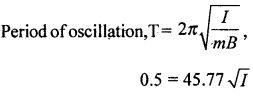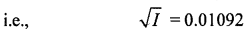or I = 1.193 × 10-4 kgm 2
∴ M.I of the coil about an axis passing through the centre and perpendicular to the plane of the coil = 1.193 × 10-4 kg m2.

Question 10.
A long straight horizontal cable carries a current of 2.5A in the direction 10° south of west to 10° north of east. The magnetic meridian of the plane happens to be 10° west of the geographic meridian. The earth’s magnetic field at the location is 0.33G, and the angle of dip is zero. Locate the line of neutral points.
Given : I = 2.5 A, BE = 0.33G, θD = 0
We know that, BH = BH for θD = 0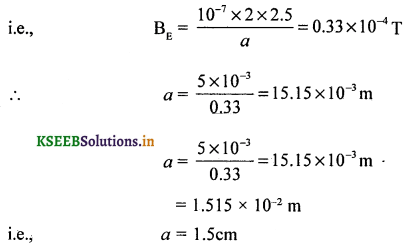Neutral poii.t will be away from the cable and above it, at a height of 1.5cmQuestion 11.
A telephone cable at a place has four long straight horizontal wires carrying a current of 1.0 A in the same direction east to west. The Earth’s magnetic field at the place is 0.39G, and the angle of dip is 35°. The magnetic declination is nearly zero. What are the resultant magnetic fields at points 4.0 cm above and below the cable?
Magnetic field at a point due to 4 wires,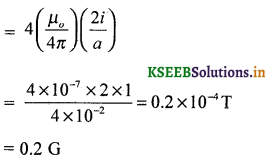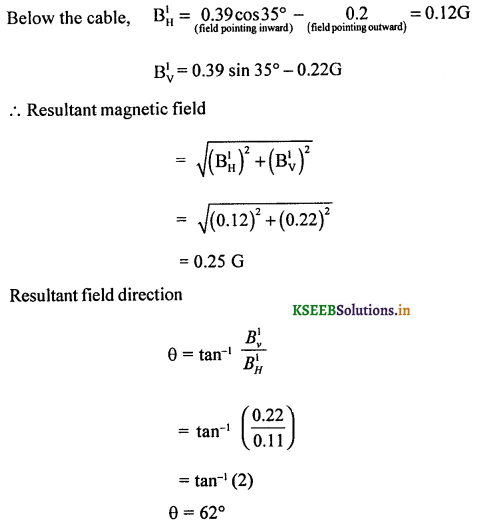Question 12.
A monoenergetic (18 keV) electron beam initially in the horizontal direction is subjected to a horizontal magnetic field of 0.04 G normal to the initial direction. Estimate the up or down deflection of the beam over a distance of 30 cm (mc = 9,11 × 10-31kg).
Given : (K.E)e = 18 × 103 × 1.6 × 10-19 J = 28.8 × 10-16 J.
BH = 0.04G = 4 × 10-6T.
Since electrons describe circular pathUp or down deflection = R(1 – cosθ)
= 11.3 (1 – 0.9996)
= 0.00398 m
= 3.98 × 10-3 m
≈ 4.0 mm

Question 13.
A sample of a paramagnetic salt contains 2.0 × 1024 atomic dipoles each of dipole moment 1.5 × 10-23 JT-1. The sample is placed under a homogeneous magnetic field of 0.64 T and cooled to a temperature of 4.2 K. The degree of magnetic saturation achieved is equal to 15%. What is the total dipole moment of the sample for a magnetic field of 0.98 T and a temperature of 2.8K? (Assume Curie’s law)
Total dipole moment = 0.15 × 1.5 × 10-23 × 2.0 × 1024 = 4.5 Am2 or JT-1
Using Curie’s law, µ ∝ B/T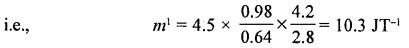New magnetic moment = 10.3 J T-1 or Am2Question 14.
A magnetic needle has a magnetic moment 5 × 10-2 Am2. If the moment of inertia of a magnet about an axis passing through the centre and perpendicular to the length of a magnet is 1.0 × 10-7 kgm2, then calculate the magnitude of the magnetic field which sets the magnet into oscillation at a frequency of 2Hz.